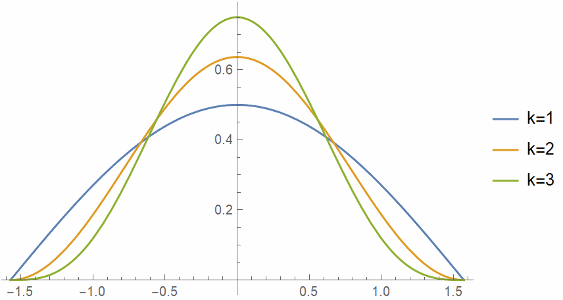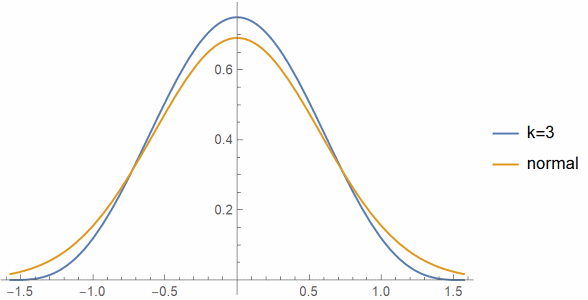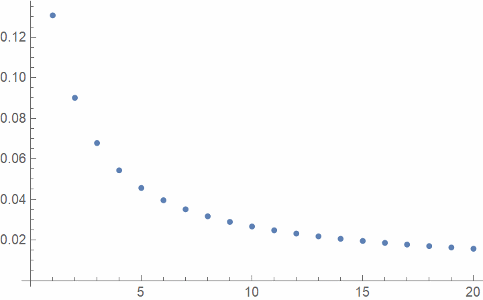# Cosine power approximation to normal

Ten years ago I wrote about how cosine makes a decent approximation to the normal (Gaussian) probability density. It turns out you get a much better approximation if you raise cosine to a power.

If we normalize cosk(t) by dividing by its integralwe get an approximation to the density function for a normal distribution with mean 0 and variance 1/k.

Here are the plots of cosk(t), normalized to integrate to 1, for k = 1, 2, and 3.And here’s a plot of cos3(t) compared to a normal with variance 1/3.And finally here’s a plot of L² error, the square root of the integral of the square of the approximation error, as a function of k.Update: You can do much better if you take a convex combination of cosine with 1 and allow non-integer powers. See this post.

## 2 thoughts on “Cosine power approximation to normal”

1. Nathan Hannon

This is somewhat interesting because normal distributions are all rescalings of one another, but powers of cosine clearly are not.

Which value of k gives the best approximation? The L^2 error seems to be strictly decreasing, but that reflects the effect of scaling; expanding the x axis by a factor of k, but shrinking the y axis by the same factor, reduces the L^2 norm of a function by sqrt(k), so it would be more natural to plot the L^2 error times sqrt(k) if you want to compare apples to apples.

2. François

If you normalize the cosine power so that it has unit variance, i.e. take cos(x/sqrt(p))^p, one can show that in the limit p->inf one recovers the normal probability density (up to the 1/sqrt(2pi) constant).